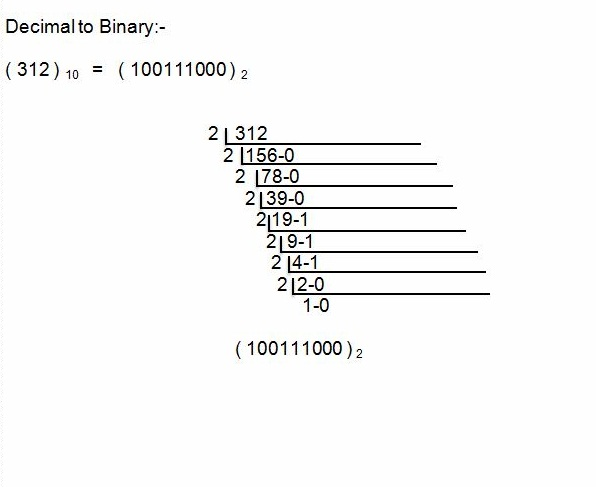# Basic idea about binary to decimal and decimal to binary

Introductions:

Basic idea about binary to decimal and decimal to binary.This is the awesome kingdom of a Computer. This is not a tips but get an Basic idea about binary to decimal and decimal to binary. It can helps you what is the binary and what is decimal. This is the mathematics term. The simple two digits 0 1 is called binary and the ten digits 0 1 2 3 4 5 6 7 8 9 are called decimal.

For example:

## Basic idea about binary to decimal and decimal to binary

When you are a student of computer science and engineering (CSE) or diploma in computer science (DCS), you have to need that idea and you can learn more about the digital magic.

See the images and get the basic idea about binary to decimal and decimal to binary.

### Basic idea about binary to decimal and decimal to binary

Binary to Decimal:-

( 100111000 )   =   ( 312 ) 10### Basic idea about binary to decimal and decimal to binary

Decimal to Binary:-

( 312 ) 10   =   ( 100111000 )  2If the tips are helpful for you, so please send us a feedback from interpersonal communication or comments us from below for our best impression. It takes just some seconds.